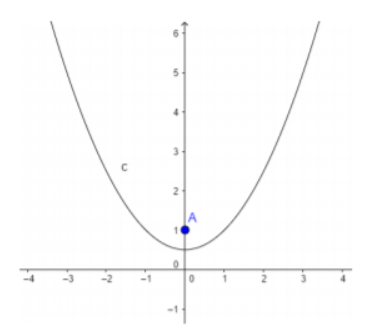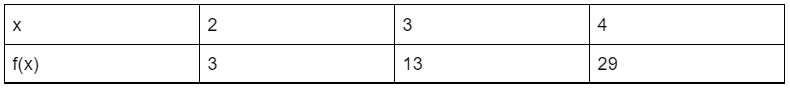# Modeling Data with Quadratic Functions

 Would You Rather Listen to the Lesson?In algebra 2, students learn the standard form of quadratic functions and how to differentiate between linear and quadratic functions and become proficient in modeling data with quadratic functions.

Learning modeling data with quadratic functions can sometimes seem challenging for students. Luckily, there are ways in which teachers can make this process as smooth as possible. So if you’re teaching this, here are some awesome teaching tips to get you through!

## Tips for Teaching Modeling Data with Quadratic FunctionsYou can start your lesson on modeling data with quadratic functions by defining quadratic functions. Explain to students that we call all functions that can be written in this form: f(x) = ax² + bx + c quadratic functions.

…where a is not a zero, and ax² is a quadratic term, bx is a linear term, and c is a constant term.

A quadratic function is basically a function in which the highest degree term is of the second degree, in contrast to linear functions, where the highest degree term is of the first degree.

Provide a few examples and explain whether they are quadratic functions, and why, such as:

• f(x) = – x² + 2x + 3 – this is a quadratic function because the highest degree term is of the second degree (- x²), where a = -1, b = 2 and c = 3
• f(x) = 7x² – x – 1 – this represents a quadratic function because the highest degree term is of the second degree (7x²), where a = 7, b = -1 and c = – 1
• f(x) = x² – even though it may not be obvious immediately, this is also a quadratic function, because the highest degree term is of the second degree (x²), where a = 1, b = 0 and c = 0
• f(x) = 2x + 1 – this represents a linear function, not a quadratic one, because the highest degree term is of the first degree

#### Example 1:

Write a function on the whiteboard and ask students to determine whether the given function is a quadratic one. For instance, you can write the following function:

f(x) = (x -1)(x – 2)

Point out that by multiplying, we’ll obtain the following:

f(x) = x² – 2x – x + 2

We’ll simplify it further by combining like terms:

f(x) = x² – 3x + 2

At this point, ask students whether this is a quadratic function. They should be able to easily observe that the highest degree term is of the second degree, meaning that the function is a quadratic one.

#### Example 2:

Write another function on the whiteboard and ask students to determine whether this represents a quadratic function. For example, you can write this function:

f(x) = x(x – 1) – x² – x

Point out that by multiplying, we’ll get the following:

f(x) = x² – x – x² – x

f(x) = – 2x

Now ask students to observe the simplified form of the function, that is, f(x) = – 2x and determine if this is a quadratic function. Students should be able to easily spot that this is not a quadratic function, but a linear one, as the highest degree term is of the first degree.

### Graph of a Quadratic Function

Explain to students that the graph of a quadratic function is a vertical parabola. Provide a drawing on the whiteboard to illustrate this parabola, such as:### How to Write a Quadratic Function from Data

Explain to students that when we have a data set and we need to find the quadratic function that represents the data, we simply need to find the coefficients a, b, and c in f(x) = ax² + bx + c. Provide an example to illustrate this process of modeling data with quadratic functions.

For example, let’s say we’re asked to find a quadratic function representing the data set in this table:Point out to students that we are given values for x and f(x). You can also remind students that f(x) is the same thing as y. The first thing to do is look at the standard form of the quadratic function, f(x) = ax² + bx + c, and try to find a, b and c, by replacing the values for x and y ( f(x).

This would result in the following for a, b and c:

a) f(2) = 3, meaning that when x is 2 in the above table, we can observe that f(x) is 3.

By replacing x, i.e. 2 in f(x) = ax² + bx + c, we’ll get: 4a + 2b + c = 3

b) f(3) = 13, in other words, when x is 3, f(x) is 13. By plugging in the value for x, i.e. 3, in f(x) = ax² + bx + c, we’ll obtain: 9a + 3b + c = 13

3) f(4) = 29, which means that when x is 4, f(x) is 29. By replacing the value for x (=4) in f(x) = ax² + bx + c, we’ll have: 16a + 4b + c = 29.

Point out that if we solve these algebraically, we’ll get the following values for a, b, and c:

a = 3

b = – 5

c = 1

This means that we can now replace the values for a, b, and c in f(x) = ax² + bx + c and transform it into f(x) = 3x² – 5x + 1.

If you have the technical possibility, you can also enrich your lesson on modeling data with quadratic functions with multimedia resources, such as different videos. Below there are a few examples of such videos.

For instance, use this video to introduce quadratic functions, as well as this video to introduce quadratic graphs. Finally, use this video to illustrate to students how to write a quadratic function from data, that is, how to model data with quadratic functions.

## Activities to Practice Modeling Data With Quadratic Functions

### Pair Work

This activity will help students practice identifying whether a given function is linear or quadratic, as well as modeling data with quadratic functions. To use this activity in your classroom, make sure to print out this Assignment Worksheet (Members Only).

Pair students up and hand out the worksheets. Explain to students that the worksheet contains several exercises related to quadratic functions. Students should work together to solve the exercises in the worksheets.

Provide a few minutes for students to complete the exercises and then open space for a discussion and reflection. How did students determine if a function is quadratic or not? Which steps did they take to write a quadratic function from the given data?

## Before You Leave…

If you like these teaching tips on modeling data with quadratic functions, and you’re looking for more algebra 2 resources, make sure to sign up for our emails to receive loads of free lessons and content!

In addition, you can sign up for a membership on MathTeacherCoach or head over to our blog, where you’ll find more math materials for kids of all ages. You’ll discover that with the resources we offer, teaching math has never been easier!